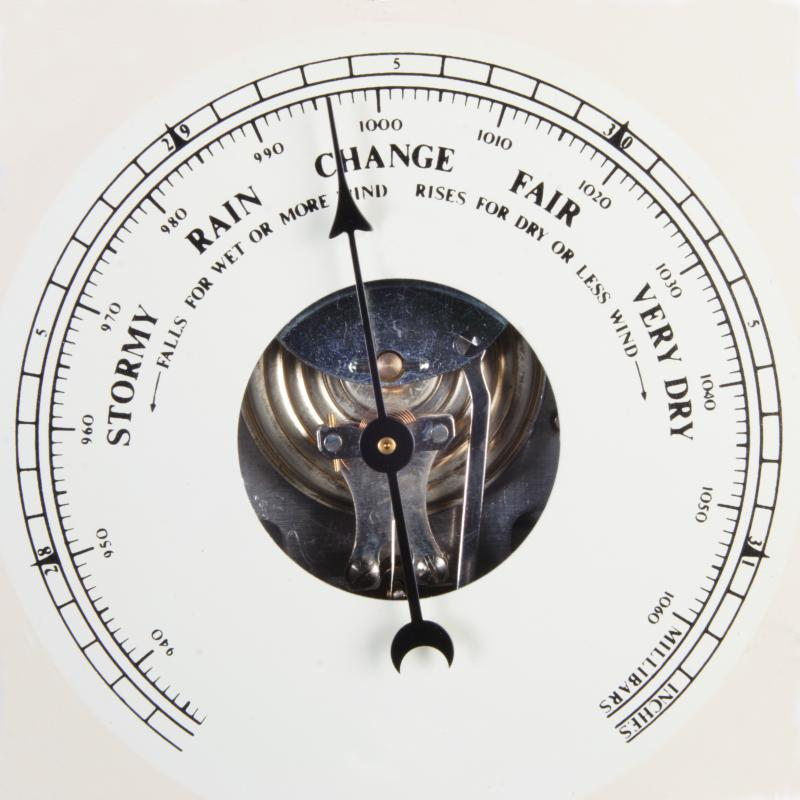What Is Absolute Pressure?

C.H. Seman
C.H. SemanAbsolute pressure is the sum of atmospheric pressure, which may be gauged using a manometer, and the gauge pressure of fluid.

In engineering, absolute pressure is the pressure of a system relative to the pressure of an absolute vacuum. In more practical terms, it is often expressed as the sum of the pressure of the atmosphere and the gauge pressure of a fluid. It is necessary in engineering calculations such as the Ideal Gas Law.

The most common expression of absolute pressure defines it as the sum of a system's gauge, or measured, pressure and the pressure of the atmosphere. The expression takes the form Pabsolute = Pgauge + Patmospheric. Atmospheric pressure is defined as the pressure of the surrounding air at or near the surface of the Earth. This pressure is not a fixed or constant value and it may vary with temperature or elevation.

Gauge pressure represents the pressure of the system as measured by a pressure measurement device. These devices, or gauges, can be classified by the ways they measure pressure. The most common types are elastic-element gauges, liquid-column gauges and electrical gauges. Unless otherwise noted by the manufacturer, most gauges do not include the pressure of the atmosphere in their readings.

In a typical chemical plant environment, absolute pressure and gauge pressure do not represent the same thing and different notations must be used to keep them separate. A common method of doing this is to add the letter a after the pressure unit to signify absolute pressure and the letter g after the pressure unit to signify gauge pressure. For example, an absolute pressure of 100 psi would become 100 psia. Similarly, a gauge pressure of 5 kPa would be 5 kPag. The U.S. National Institute of Standards and Technology, however, prefers the clarifying letter to be applied not to the unit but to the letter P. For example, Pg = 25 kPa would be preferable to P = 25 kPag.

This measurement of pressure is most commonly used in engineering calculations such as the Ideal Gas Law. When performing such equations, engineers must use the correct pressure to avoid costly mistakes or dangerous operation. The difference in absolute and gauge pressure is much more noticeable at pressures in which the atmospheric pressure is of the same order of magnitude as the gauge pressure.

The error in neglecting the atmospheric component of absolute pressure can be demonstrated by examining a closed cylinder of an ideal gas with a temperature of 77° Fahrenheit (25° Celsius) and a volume of 1.0 m3. If the pressure gauge on the cylinder reads 100 kPa and the pressure of the atmosphere is not considered, then the calculated number of moles of gas in the cylinder is approximately 40.34. If the pressure of the atmosphere also is 100 kPa, then the absolute pressure is actually 200 kPa and the correct number of moles is 80.68. The actual number of moles is twice the amount in the original calculation, demonstrating the importance of using the correct pressure.

You might also Like